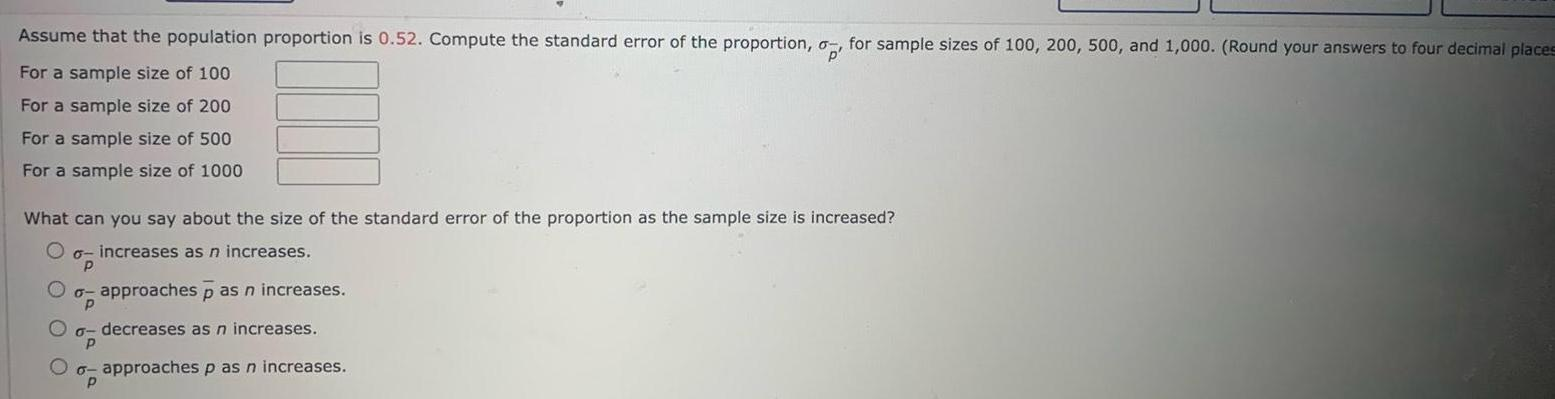Question:

# Assume that the population proportion is 0 52 Compute the

Last updated: 11/21/2023Assume that the population proportion is 0 52 Compute the standard error of the proportion o for sample sizes of 100 200 500 and 1 000 Round your answers to four decimal places For a sample size of 100 For a sample size of 200 For a sample size of 500 For a sample size of 1000 What can you say about the size of the standard error of the proportion as the sample size is increased Oo increases as n increases p o approaches p as n increases p decreases as n increases Oo approaches p as n increases P p